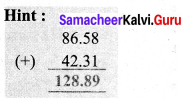Students can Download Maths Chapter 1 Number System Ex 1.2 Questions and Answers, Notes Pdf, Samacheer Kalvi 7th Maths Book Solutions Guide Pdf helps you to revise the complete Tamilnadu State Board New Syllabus and score more marks in your examinations.

## Tamilnadu Samacheer Kalvi 7th Maths Solutions Term 3 Chapter 1 Number System Ex 1.2

Question 1.
Add by using grid 0.51 + 0.25.
Solution:
Here 0.51 = $$\frac { 51 }{ 100 }$$ and 0.25 = $$\frac { 25 }{ 100 }$$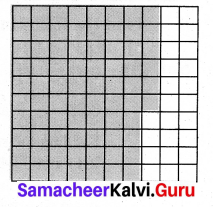First we shade the region 0.51 and then 0.25.
The sum is the total shaded area. 0.51 + 0.25 = 0.76

Question 2.
Add the following by using place value grid.
(i) 25.8 + 18.53
(ii) 17.4 + 23.435
Solution:
(i) 25.8 + 18.53.
Using place value grid.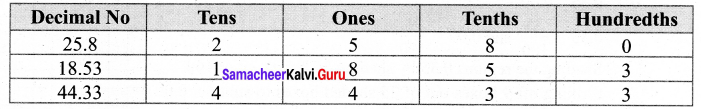Therefore 25.8 + 18.53 = 44.33

(ii) 17.4 + 23.435
Lets use the place value grid.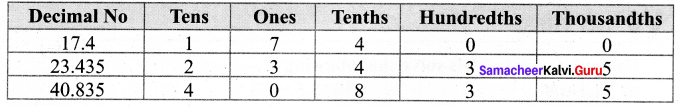Therefore 17.4 + 23.435 = 40.835Question 3.
Find the value of 0.46 – 0.13 by grid model.
Solution:
Here 0.46 = $$\frac { 46 }{ 100 }$$ and 0.13 = $$\frac { 13 }{ 100 }$$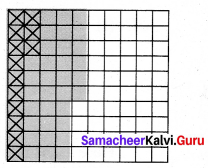Shading the region 0.46 and then crossing out 0.13 from the shaded area. The left out shaded region without cross marks is the difference. So 0.46 – 0.13 = 0.33

Question 4.
Subtract the following by using place value grid, (i) 6.567 from 9.231 (ii) 3.235 from 7
Solution:
(i) Let as use place value grid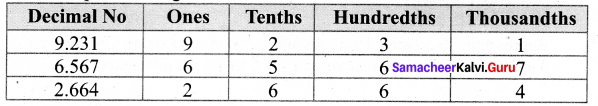Therefore 9.231 – 6.567 = 2.664

(ii) Let as use place value grid.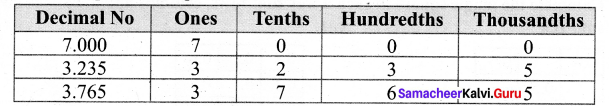Therefore 7 – 3.235 = 3.765Question 5.
Simplify: 23.5 – 27.89 + 35.4 – 17.
Solution:
23.5 – 27.89 + 35.4 – 17 = 14.01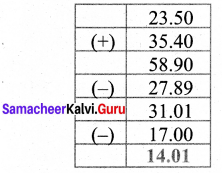Question 6.
Sulaiman bought 3.350 kg of Potato, 2.250 kg of Tomato and some onions. If the weight of the total items are 10.250 kg, then find the weight of onions?
Solution:
Weight of Potato = 3.350 kg
Weight of Tomato = 2.250 kg
Total weight of Potato and Tomato = (3.350 + 2.250 kg)
= 5.600 kg
Weight of potato, tomato and onions = 10.250
Weight of potato and tomato = 5.600
∴ Weight of onions = (10.250 – 5.600) Kg = 4.650 Kg
Weight of onions = 4.650 KgQuestion 7.
What should be subtracted from 7.1 to get 0.713?
Solution:
To get the number to be subtracted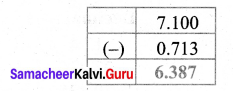We have 7.1 – 0.713 = 6.387
∴ The number to be subtracted = 6.387

Question 8.
How much is 35.6 km less than 53.7 km?
Solution:
To get the answer we must subtract 53.7km – 35.6 km = 18.1 km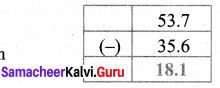So 35.6 km is 18.1 km less than 53.7 km.

Question 9.
Akilan purchased a geometry box for ₹ 25.75, a pencil for ₹ 3.75 and a pen for ₹ 17.90. He gave ₹ 50 to the shopkeeper. What amount did he get back?
Solution:
Cost of geometry box = ₹ 25.75 (+)
Cost of Pencil box = ₹ 3.75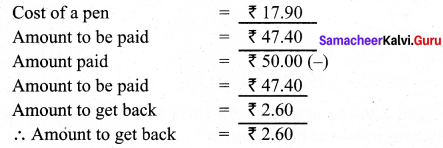Question 10.
Find the perimeter of an equilateral triangle with a side measuring 3.8 cm.
Solution:
Perimeter of an equilateral triangle = (Side + Side + Side) Sq. units.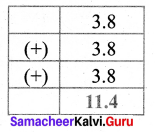Given side = 3.8
∴ Perimeter = 3.8 + 3.8 + 3.8
Perimeter of the triangle = 11.4 cm

Objective Type Questions

Question 1.
1.0 + 0.83 = ?
(i) 0.17
(ii) 0.71
(iii) 1.83
(iv) 1.38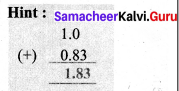(iii) 1.83Question 2.
7.0 – 2.83 = ?
(i) 3.47
(ii) 4.17
(iii) 7.34
(iv) 4.73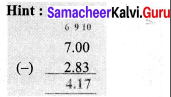(ii) 4.17

Question 3.
Subtract 1.35 from 3.51
(i) 6.21
(ii) 4.86
(iii) 8.64
(iv) 2.16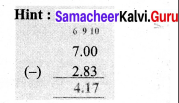(iv) 2.16

Question 4.
Sum of two decimals is 4.78 and one decimal is 3.21 then the other one is
(i) 1.57
(ii) 1.75
(iii) 1.59
(iv) 1.58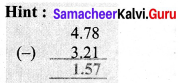(i) 1.57Question 15.
The difference of two decimals is 86.58 and one of the decimal is 42.31 Find the other one
(i) 128.89
(ii) 128.69
(iii) 128.36
(iv) 128.39